# Symbolic Computation and More with Multivariate Polynomials

Symbolic computing with multivariate polynomials in R.## Specifying polynomials

mpoly is a simple collection of tools to help deal with multivariate polynomials symbolically and functionally in R. Polynomials are defined with the `mp()` function:

Term orders are available with the reorder function:

Vectors of polynomials (`mpolyList`’s) can be specified in the same way:

## Polynomial parts

You can extract pieces of polynoimals using the standard `[` operator, which works on its terms:

There are also many other functions that can be used to piece apart polynomials, for example the leading term (default lex order):

You can also extract information about exponents:

## Polynomial arithmetic

Arithmetic is defined for both polynomials (`+`, `-`, `*` and `^`)…

… and vectors of polynomials:

## Some calculus

You can compute derivatives easily:

## Function coercion

You can turn polynomials and vectors of polynomials into functions you can evaluate with `as.function()`. Here’s a basic example using a single multivariate polynomial:

Here’s a basic example with a vector of multivariate polynomials:

Whether you’re working with a single multivariate polynomial or a vector of them (`mpolyList`), if it/they are actually univariate polynomial(s), the resulting function is vectorized. Here’s an example with a single univariate polynomial.

Here’s an example with a vector of univariate polynomials:

You can use this to visualize a univariate polynomials like this: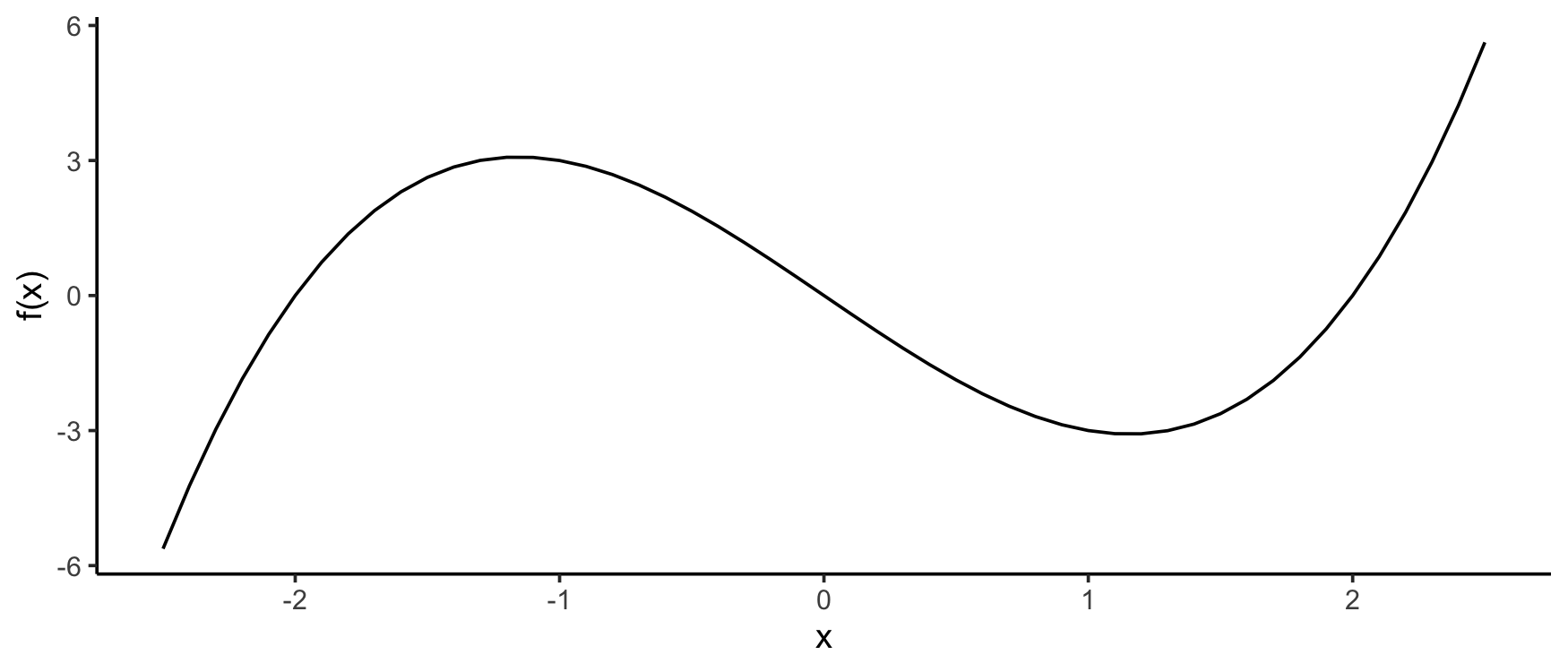For multivariate polynomials, it’s a little more complicated: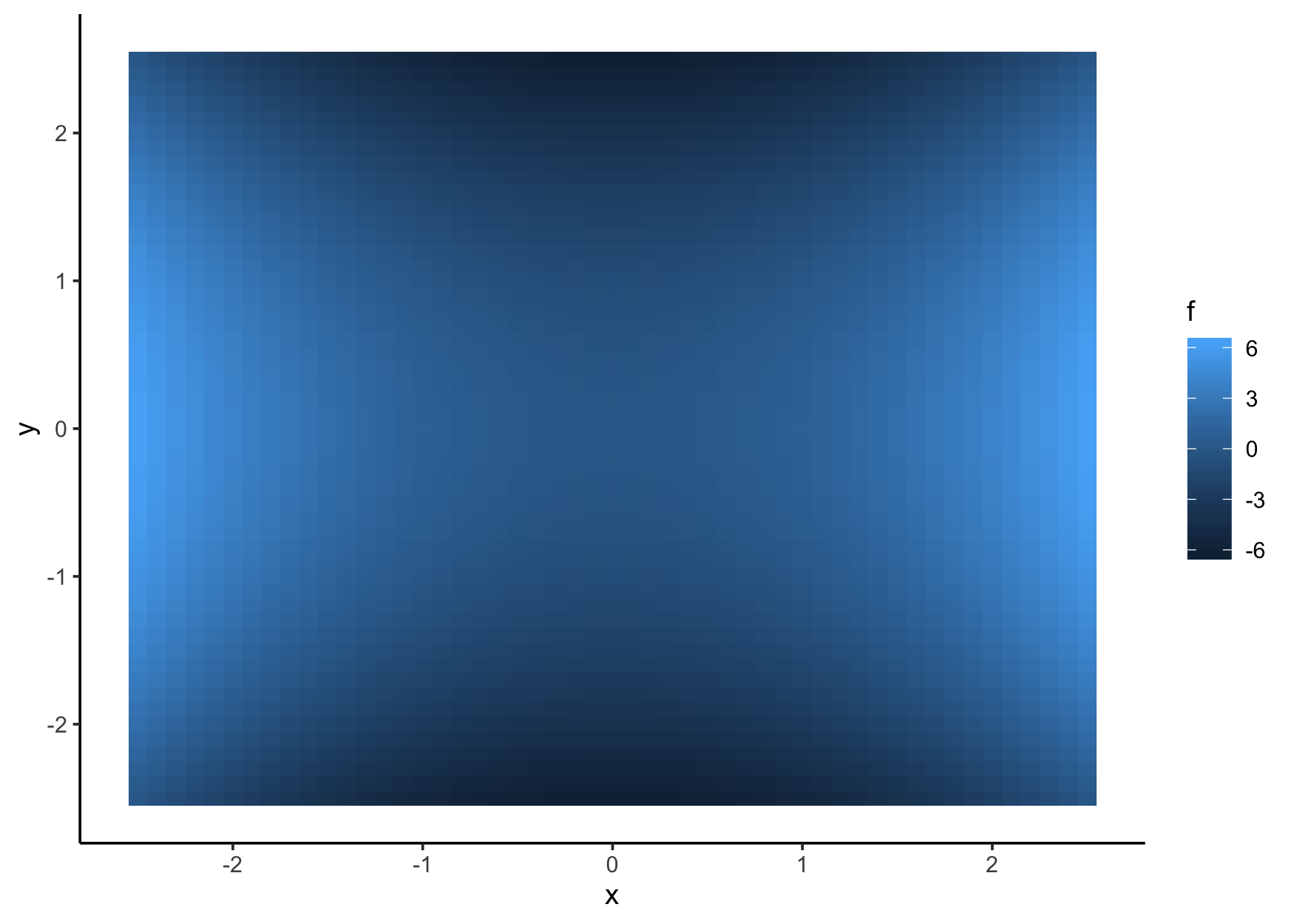## Algebraic geometry

Grobner bases are no longer implemented, see m2r

Homogenization and dehomogenization:

## Special polynomials

mpoly can make use of many pieces of the polynom and orthopolynom packages with `as.mpoly()` methods. In particular, many special polynomials are available.

#### Chebyshev polynomials

You can construct Chebyshev polynomials as follows:

And you can visualize them: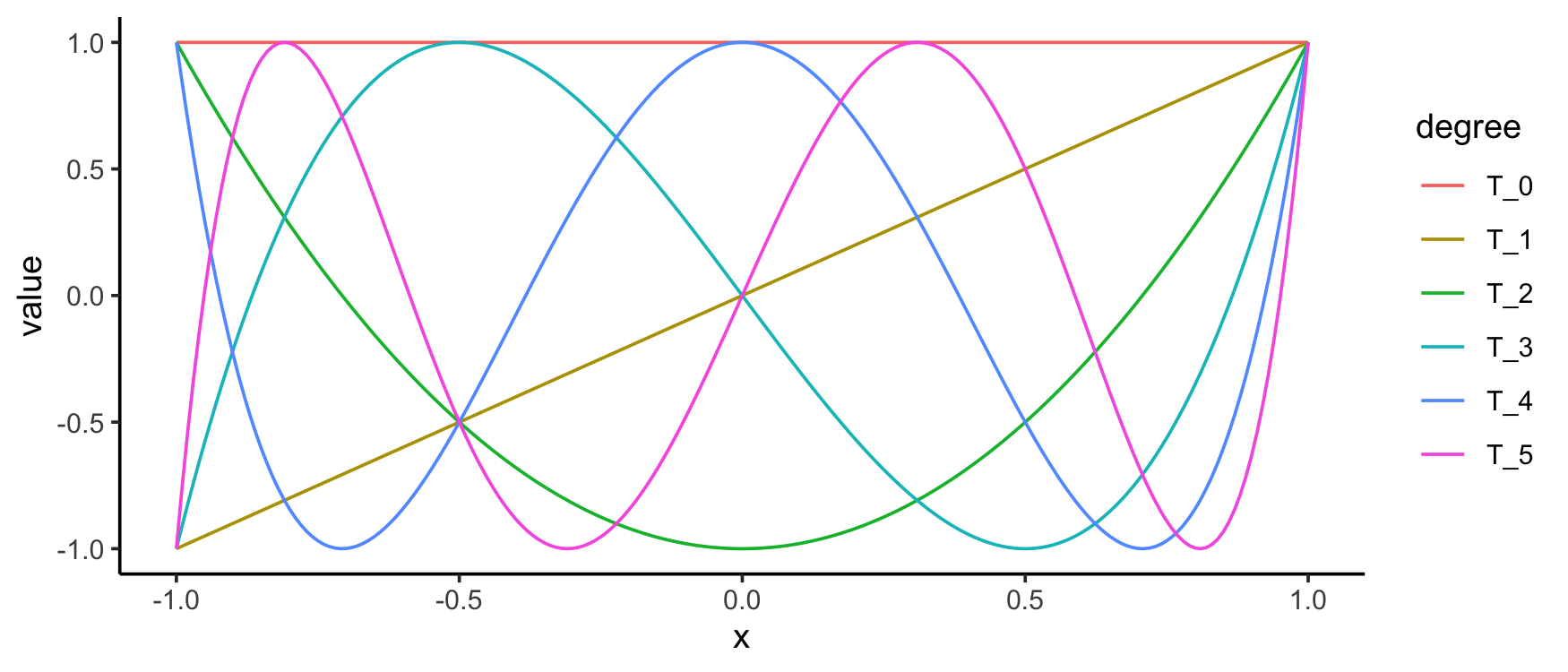#### Jacobi polynomials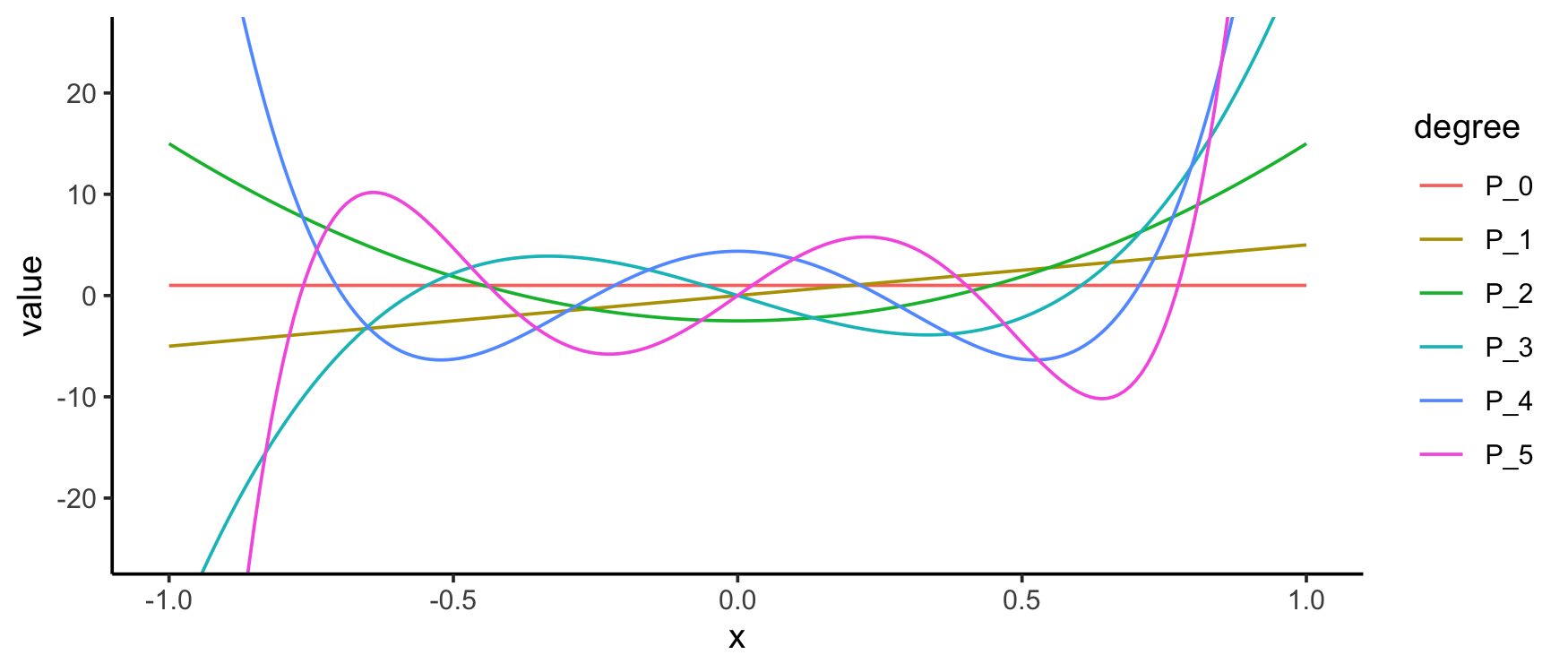#### Legendre polynomials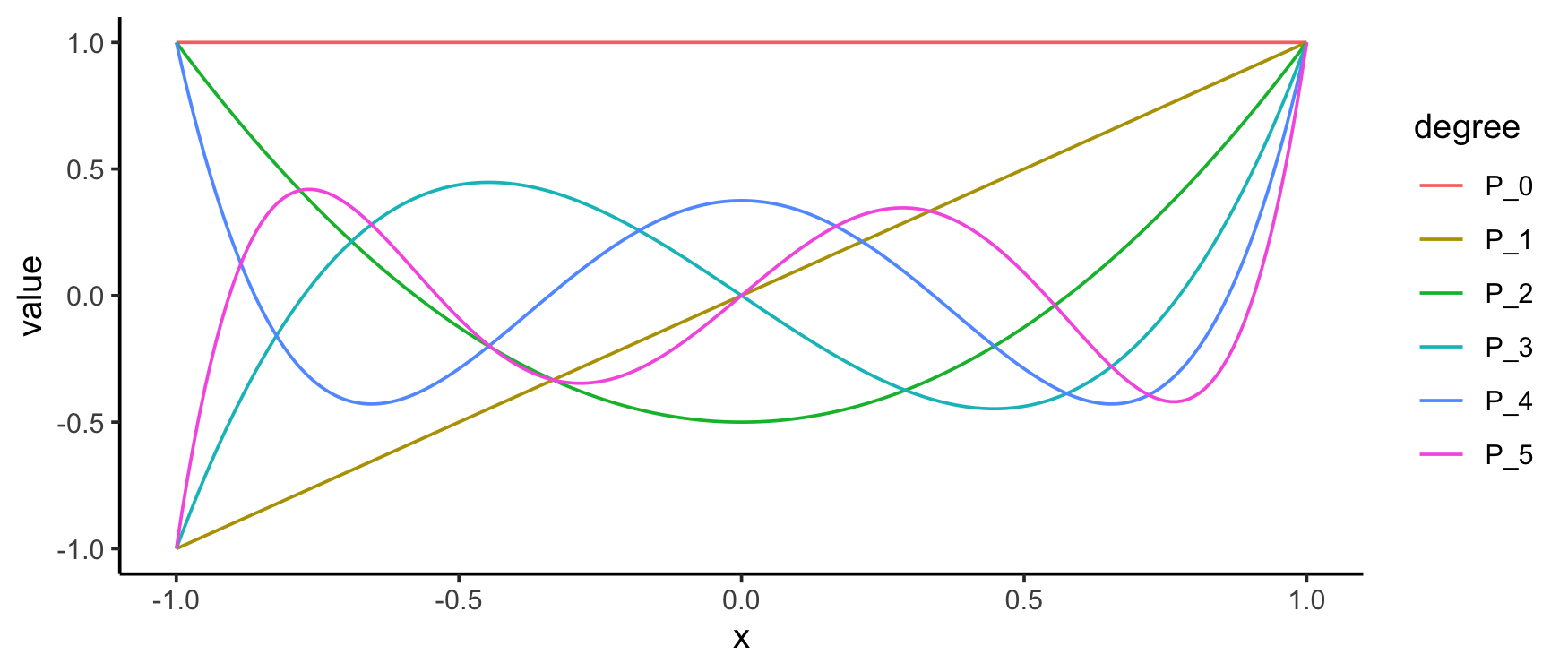#### Hermite polynomials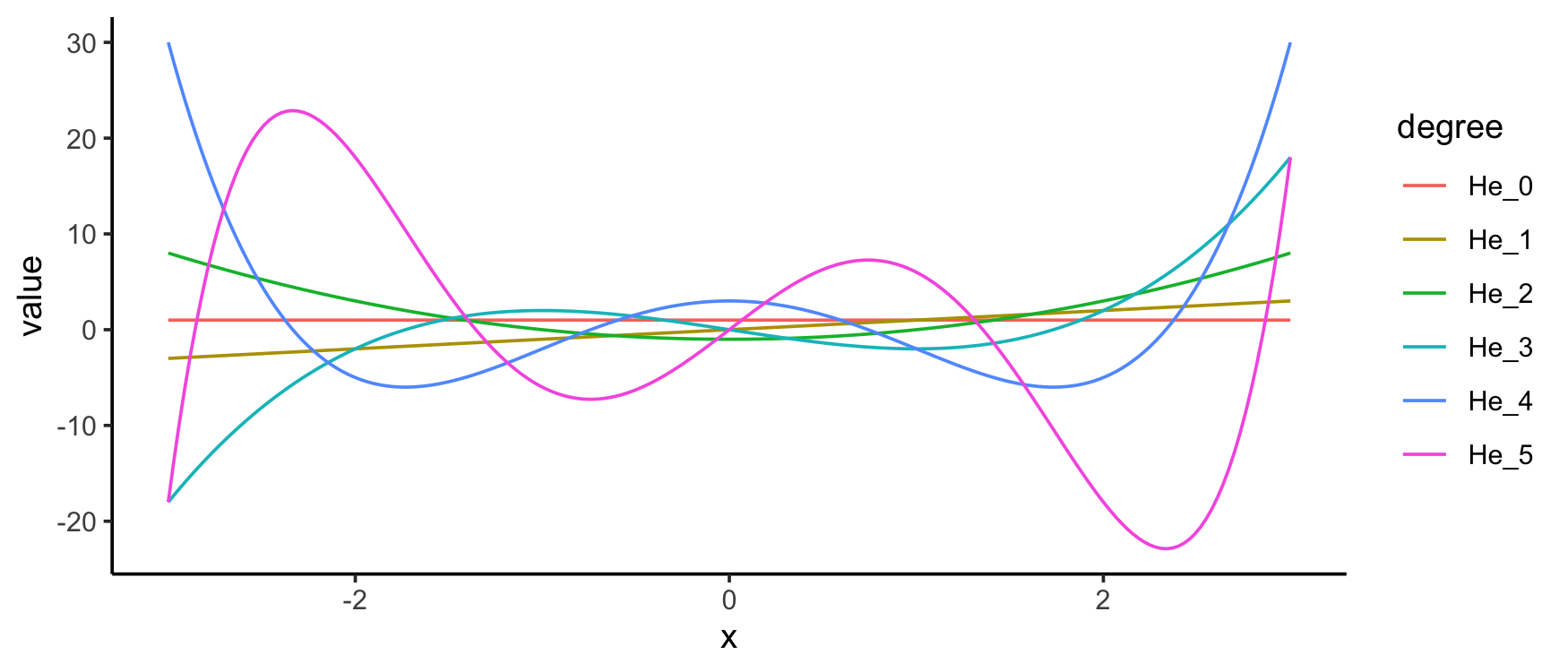#### (Generalized) Laguerre polynomials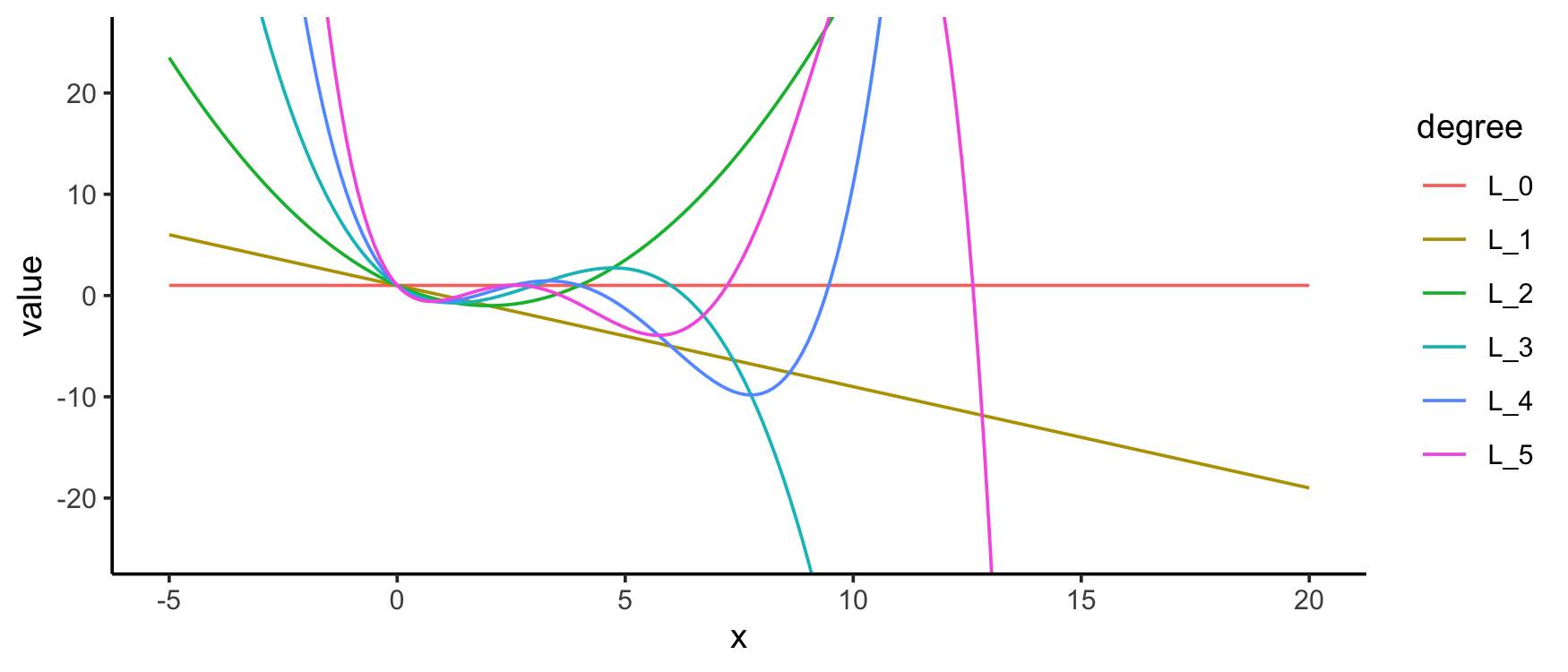#### Bernstein polynomials

Bernstein polynomials are not in polynom or orthopolynom but are available in mpoly with `bernstein()`: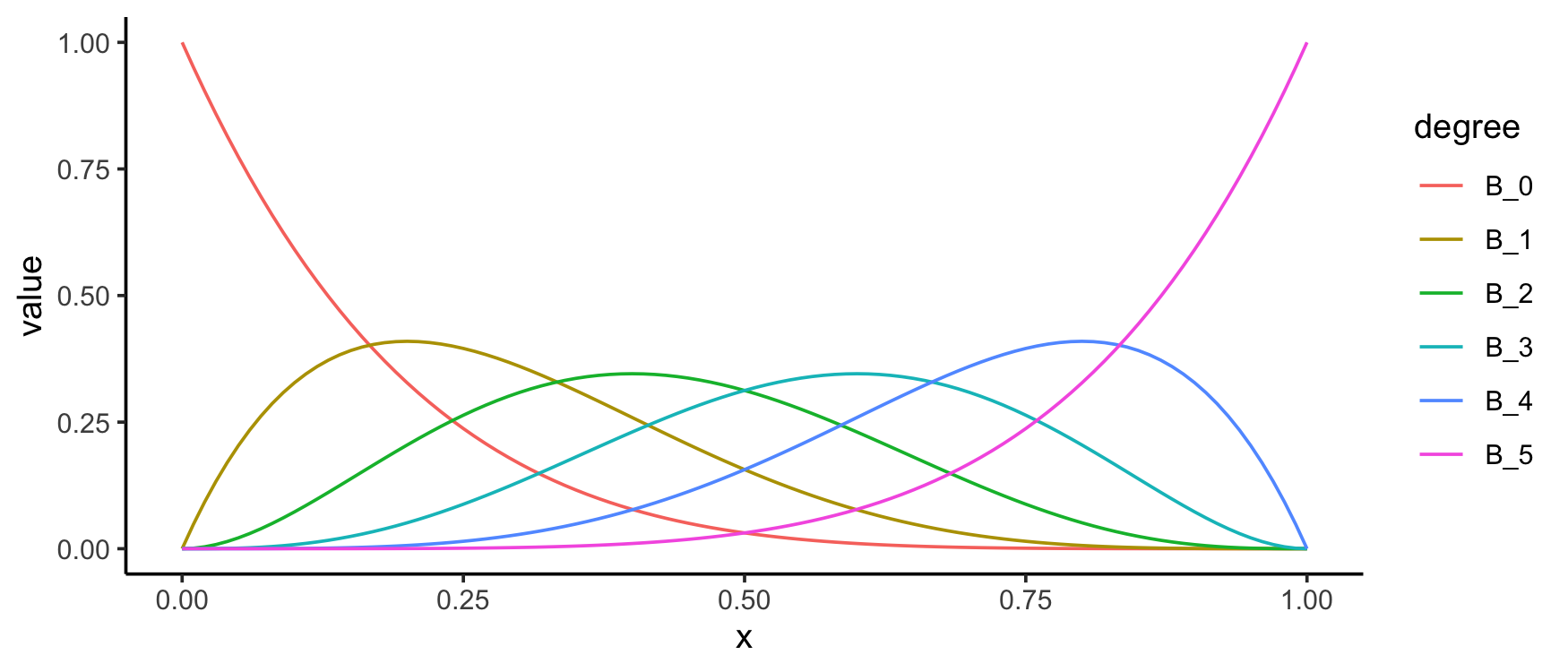You can use the `bernsteinApprox()` function to compute the Bernstein polynomial approximation to a function. Here’s an approximation to the standard normal density: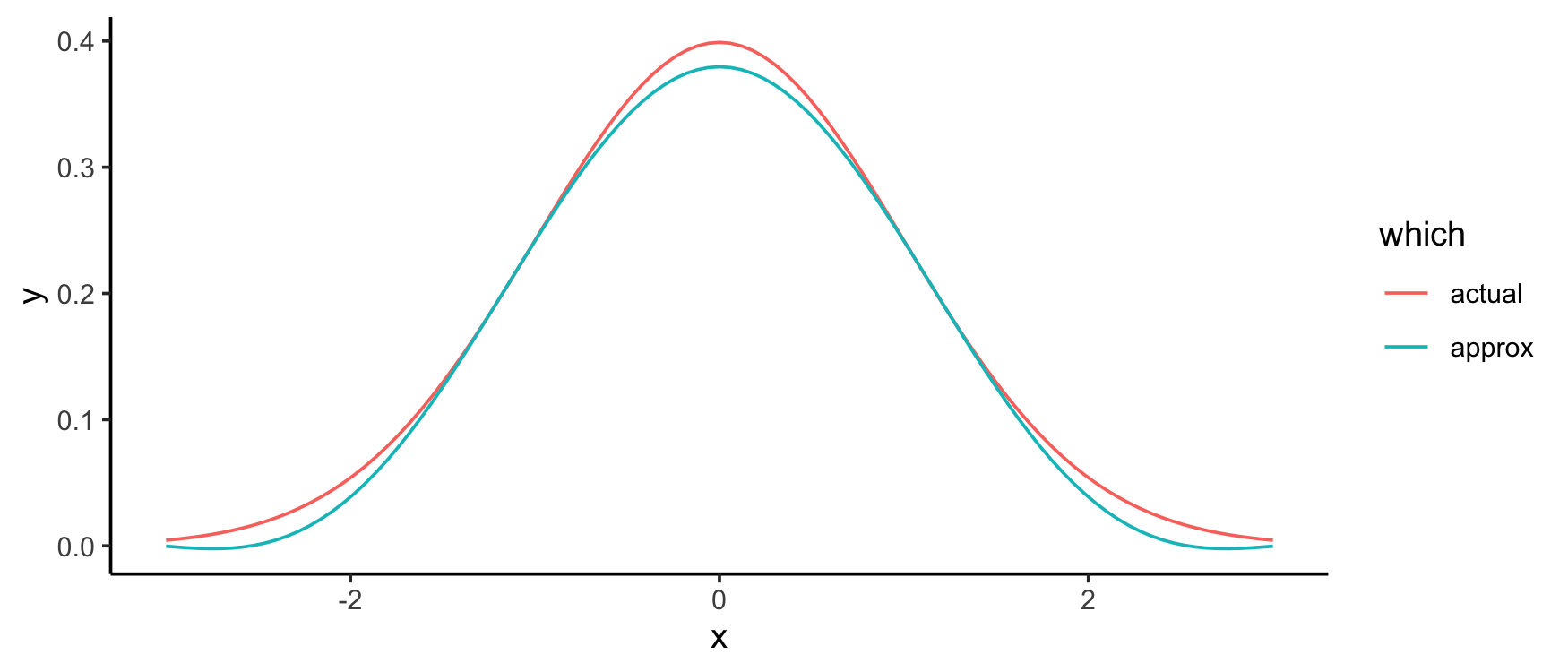## Bezier polynomials and curves

You can construct Bezier polynomials for a given collection of points with `bezier()`:

And viewing them is just as easy: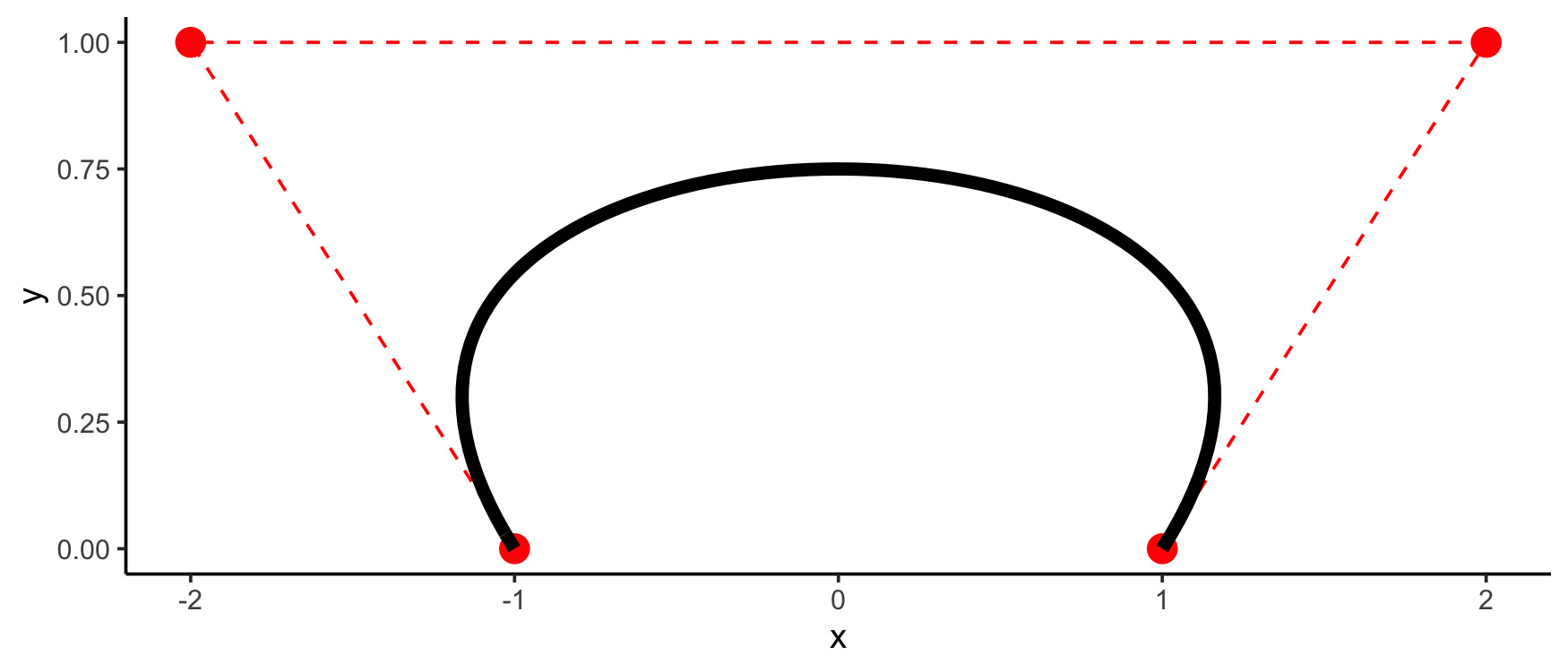Weighting is available also: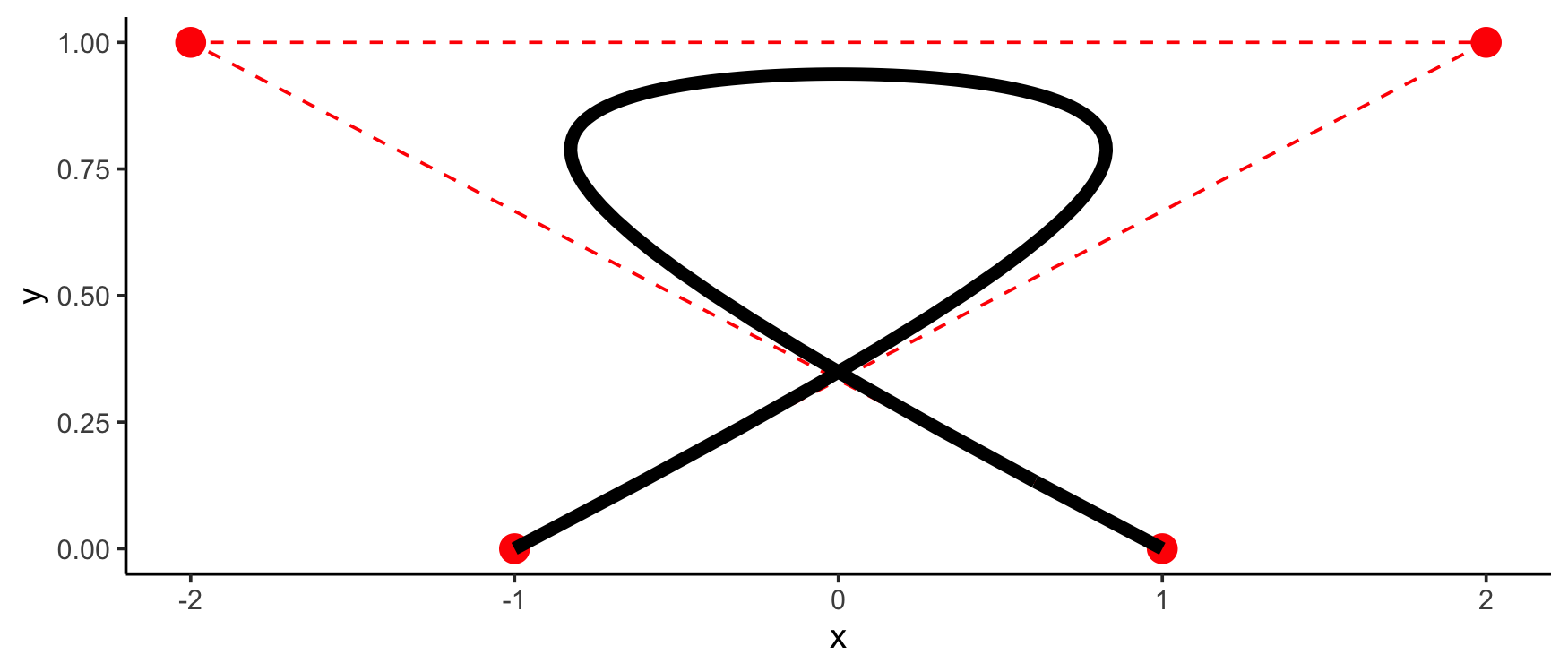To make the evaluation of the Bezier polynomials stable, `as.function()` has a special method for Bezier polynomials that makes use of de Casteljau’s algorithm. This allows `bezier()` to be used as a smoother: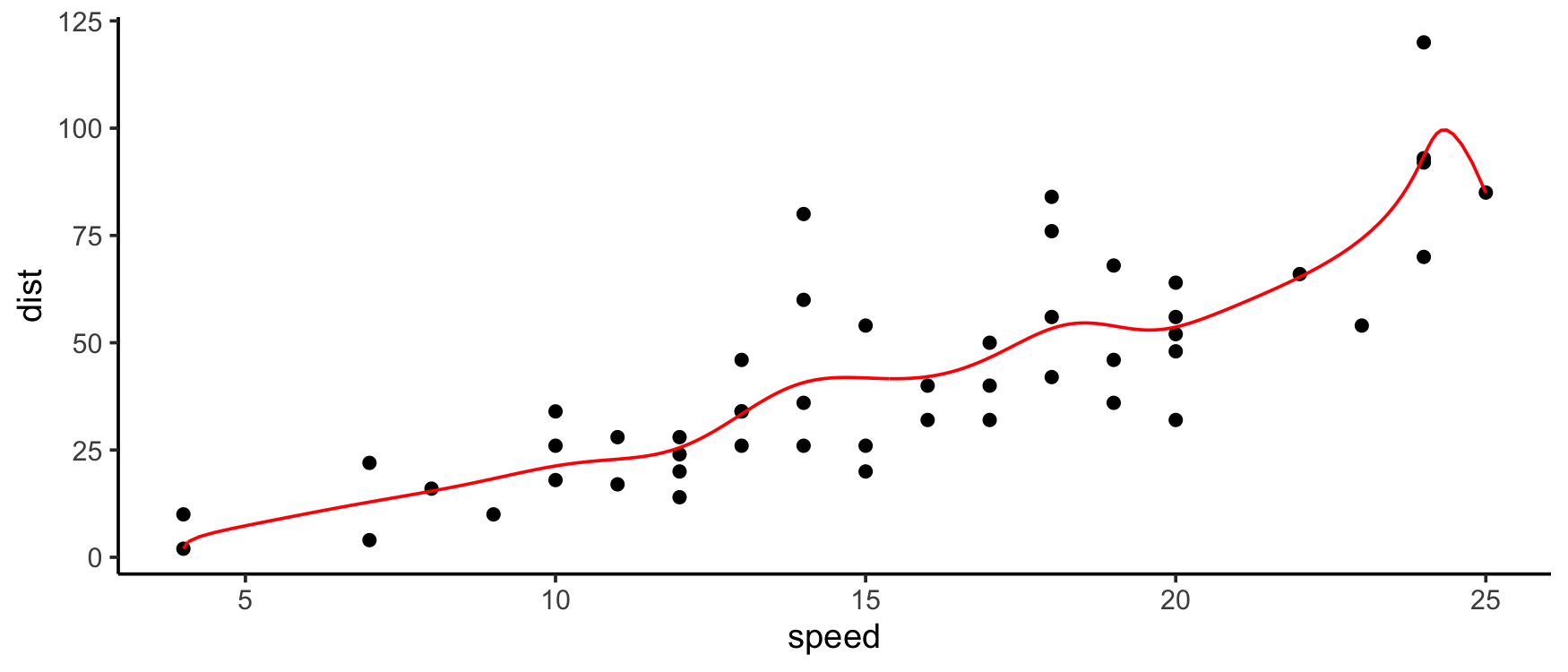## Other stuff

I’m starting to put in methods for some other R functions: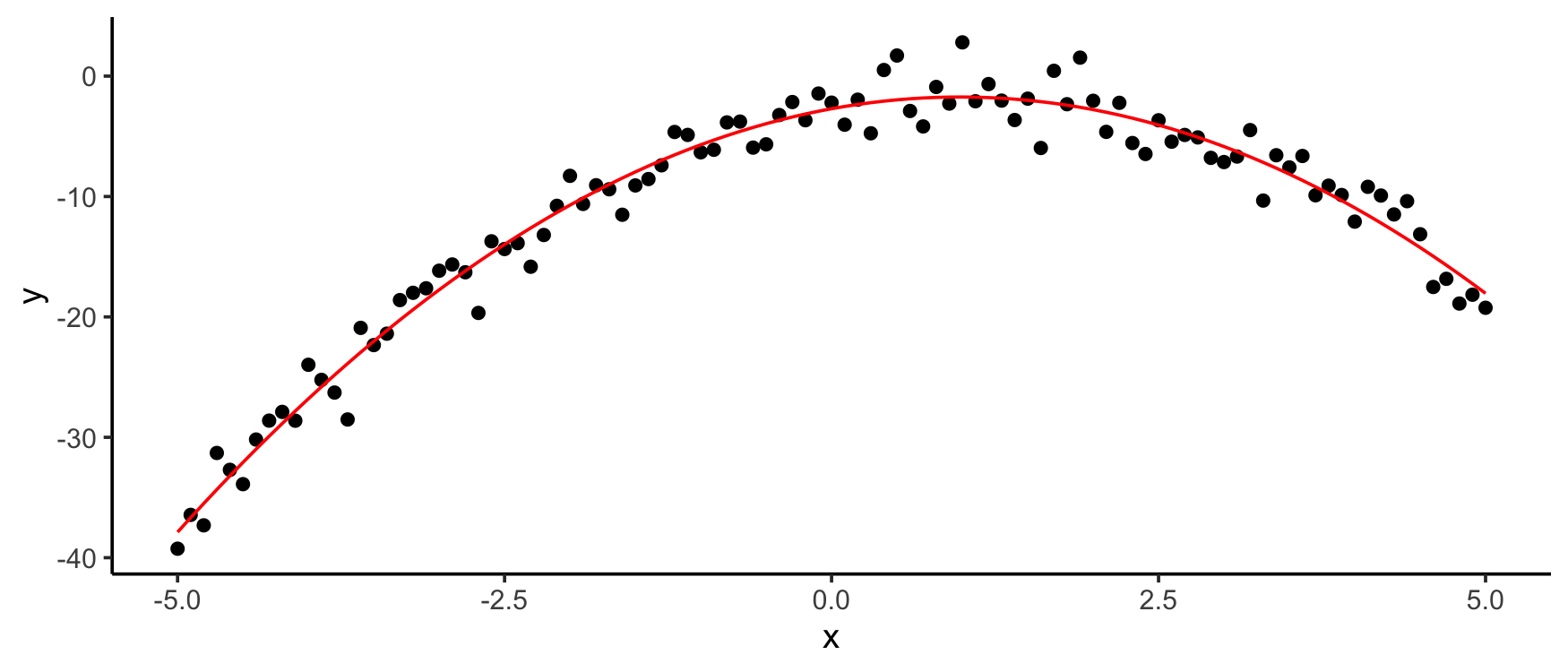## Installation

• From CRAN: `install.packages("mpoly")`

• From Github (dev version):

## Acknowledgements

This material is based upon work partially supported by the National Science Foundation under Grant No. 1622449.

# News

## Version 1.1.0

CHANGES

• Packages are now imported more nicely (@importFrom in all cases).
• mp() is now based on R's parser and mpoly arithmetic. The performance boost varies with things like how many parenthetical expressions are used, but in basic cases ranged from one to a few orders of magnitude. More importantly, many hard to catch tokenizing problems should be gone.

FIXES

• A bug in as.function() that caused it to error on univariate polynomials with indeterminates with numbers in them (e.g. x1) is now fixed. (Thanks @Blaza)

• A bug in mpoly/numeric arithmetic. Fixed by @Blaza (thanks!) and tests added.

## Version 1.0.5

NEW FEATURES

• Add is.unipoly(), a predicate for univariate polynomials.
• Add solve_unipoly(), a mpoly wrapper for polyroot().
• plug() now works on mpolyLists.

CHANGES

• as.function.mpoly() and as.function.mpolyList() no longer message when the resulting function arguments are clear.

## Version 1.0.4

FIXES

• A bug in scientific notation causing mp() to hang has been fixed. (Thanks @sommars)
• Move README from figures/ to tools/
• Add https:// for paper reference

## Version 1.0.3

NEW FEATURES

• as.mpoly() methods for lm objects using poly(, raw = TRUE) statements now works properly.

CHANGES

• the print.mpoly and print.mpolyList methods now print with cat() instead of message(). they also accept a silent argument, in which case the output is suppressed; characters are returned silently. the argument order has changed so that stars follows varorder and order.

FIXES

• a stray browser() statement was removed from as.mpoly().

## Version 1.0.2

NEW FEATURES

• you can now pass * for multiplication in mp, so that mp("x*x") works properly.

CHANGES

• all java stuff has been removed; see the m2r package for grobner basis computations.

## Version 1.0.1

NEW FEATURES

• mpoly now provided functions for extracting the leading term (LT), leading coefficient (LC), leading monomial (LM), and arbitrary term (as in p). You can also compute the multidegree of a polynomial (multideg).
• new function exponents extracts the exponents of an mpoly.
• new functions homogenize, dehomogenize, homogeneous_components extract those components of a multivariate polynomial.
• as.function() now returns vectorized functions when the polynomial(s) are only of one variable.

CHANGES

• terms.mpoly is now deprecated, use monomials.
• reorder.mpoly now defaults to the lex order.

## Version 1.0.0

CHANGES

• Versioned directly modulo very minor CRAN changes from 0.1.1 for CRAN consistency.

## Version 0.1.1

NEW FEATURES

• A new function called plug allows users to plug values into indeterminates in mpoly objects.
• mpoly now imports the polynom and orthopolynom packages, and has as.mpoly methods to parse their objects into mpoly objects.
• mpoly now imports reshape2 for some visualization stuff.
• A new function called chebyshev allows users to compute Chebyshev polynomials. This is based on orthopolynom.
• New functions called bernstein and bernsteinApprox allow users to compute Bernstein polynomials and approximate functions with Bernstein polynomials, see examples. A special as.function method exists for evaluating these in a numerically stable way.
• A new function called bezier allows users to compute the polynomials associated with Bezier curves. A special as.function method exists for evaluating these in a numerically stable way via de Casteljau's algorithm, based on a new function called bezierFunction (exported).
• Several new predicate functions are exported.
• ggplot2 is now imported.

CHANGES

• The internals of tuples have been changed, but the user shouldn't expect to see them.

FIXES

• A bug in mp functions where non-parenthetical exponents in parenthetical expressions were mis-parsed has been fixed.
• round.mpoly now drops terms rounded to have 0 coefficients.
• mpolyList now can be used inside functions and other environments (thanks @gitlongor)

## Version 0.1.0

NEW FEATURES

• is.linear function.
• round now has a method applicable to mpoly objects that rounds the coefficients. this is particularly nice in combination with as.mpoly.lm.
• Hard braces are now allowed for variables, e.g. x[1,1]

CHANGES

• Vastly enhanced mp parser based on stringr 9.0.0 (now dependent on stringi).
• Tests added.
• Description file updated for CRAN's standards.

## Version 0.0.5

NEW FEATURES

• Parenthetical expressions are now handled.

CHANGES

• Permutations now returns a better ordered listing of the permutations of a set. It also allows for the more flexible specification of (e.g.) permutations(5) for permutations(1:5).

FIXES

• Some negative parsing in mp has been fixed.

## Version 0.0.4

CHANGES

• Division symbols in mp now return an error.
• mpoly now has a GPL-2 license.

FIXES

• Fixed a glitch in print.mpoly that made mp("-1 + y") print as "-1 1 + y".
• mpolyList now works on single elements, either a mpoly object (converting it to a mpolyList) or a call to mp.
• mp now properly handles constants raised to powers.

## Version 0.0.3

FIXES

• Fixed an issue with as.mpoly (and is.mpoly.lm).

## Version 0.0.2

NEW FEATURES

• as.mpoly method now defined for lm objects which makes an mpoly object out of a linear model.

PACKAGE GENESIS

# Reference manual

install.packages("mpoly")

1.1.1 by David Kahle, a year ago

https://github.com/dkahle/mpoly

Report a bug at https://github.com/dkahle/mpoly/issues

Browse source code at https://github.com/cran/mpoly

Authors: David Kahle [aut, cre]

Documentation:   PDF Manual

Task views: Numerical Mathematics

GPL-2 license

Suggests testthat, magrittr, dplyr, covr

Imported by latte, mvp.

Depended on by m2r.

Suggested by symmoments.

See at CRAN